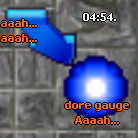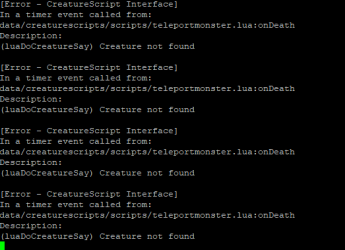# Time Top On Teleport

#### Forkz

##### Member
Hi,
I am using the system below to create teleportation when the "monster" is over and I would like it to show the time that teleportation will close.
Example:Lua:
``````local tps = {
["Abomination Fury"] = {pos = {x=1835, y=980, z=11}, toPos = {x=2135, y=1066, z=9}, time = 300},
["Orshabaal"] = {pos = {x=1835, y=980, z=11}, toPos = {x=2135, y=1066, z=9}, time = 300}
}
local function removeTp(tp)
local t = getTileItemById(tp.pos, 1387).uid
return t > 0 and doRemoveItem(t) and doSendMagicEffect(tp.pos, CONST_ME_POFF)
end
function onDeath(cid)
local tp = tps[getCreatureName(cid)]
if tp then
doCreateTeleport(1387, tp.toPos, tp.pos)
doCreatureSay(cid, "O teleport irá sumir em "..tp.time.." segundos.", TALKTYPE_ORANGE_1)
end
return true
end``````

Solution
It worked, opened the teleport, but the cooling didn't work, this error occurred in the distro

View attachment 61182View attachment 61184
Lua:
``````local tps = {
["Abomination Fury"] = {pos = {x=1835, y=980, z=11}, toPos = {x=2135, y=1066, z=9}, time = 300},
["Orshabaal"] = {pos = {x=1835, y=980, z=11}, toPos = {x=2135, y=1066, z=9}, time = 300}
}

function removeTpAndShowTime(tp, secondsRemaining, cid)
if secondsRemaining == 0 then
local t = getTileItemById(tp.pos, 1387).uid
return t > 0 and doRemoveItem(t) and doSendMagicEffect(tp.pos, CONST_ME_POFF)
end
-- if cid no longer exists, find a random player to use instead
if not isCreature(cid) then
for _, pid in ipairs(getPlayersOnline()) do
cid...``````

#### Xikini

##### I whore myself out for likes
Support Team
Use a looping function.

Lua:
``````function countdownTimer(secondsRemaining)
doCreatureSay(cid, math.floor(secondsRemaining / 60) .. ":" .. (secondsRemaining % 60), TALKTYPE_ORANGE_1)
if secondsRemaining > 0 then
end
end``````
Lua:
``````-- then in your script use this to activate the timer
local seconds = 5 * 60 -- 5 minutes in seconds
countdownTimer(seconds)``````

Of course use extra parameters to make the numbers appear on a specific position, if required.

•Forkz, Pedrinpo and Sarah Wesker

#### Sarah Wesker

##### ค∂vαηcε รүηтαx ❤
Support Team
Lua:
``````local tps = {
["Abomination Fury"] = {pos = {x=1835, y=980, z=11}, toPos = {x=2135, y=1066, z=9}, time = 300},
["Orshabaal"] = {pos = {x=1835, y=980, z=11}, toPos = {x=2135, y=1066, z=9}, time = 300}
}

function removeTpAndShowTime(tp, secondsRemaining, cid)
if secondsRemaining == 0 then
local t = getTileItemById(tp.pos, 1387).uid
return t > 0 and doRemoveItem(t) and doSendMagicEffect(tp.pos, CONST_ME_POFF)
end

cid = isCreature(cid) and cid or getPlayersOnline()
if cid then
doCreatureSay(cid, math.floor(secondsRemaining / 60) .. ":" .. (secondsRemaining % 60), TALKTYPE_ORANGE_1, false, 0, tp.pos)
end
addEvent(removeTpAndShowTime, 1000, tp, secondsRemaining - 1, cid)
end

function onDeath(cid)
local tp = tps[getCreatureName(cid)]
if tp then
doCreateTeleport(1387, tp.toPos, tp.pos)
doCreatureSay(cid, "O teleport irá sumir em "..tp.time.." segundos.", TALKTYPE_ORANGE_1)
removeTpAndShowTime(tp, tp.time, cid)
end
return true
end``````

Last edited:
•Forkz and Xikini

#### Forkz

##### Member
Lua:
``````local tps = {
["Abomination Fury"] = {pos = {x=1835, y=980, z=11}, toPos = {x=2135, y=1066, z=9}, time = 300},
["Orshabaal"] = {pos = {x=1835, y=980, z=11}, toPos = {x=2135, y=1066, z=9}, time = 300}
}

function removeTpAndShowTime(tp, secondsRemaining, cid)
if secondsRemaining == 0 then
local t = getTileItemById(tp.pos, 1387).uid
return t > 0 and doRemoveItem(t) and doSendMagicEffect(tp.pos, CONST_ME_POFF)
end

doCreatureSay(cid, math.floor(secondsRemaining / 60) .. ":" .. (secondsRemaining % 60), TALKTYPE_ORANGE_1, false, 0, tp.pos)
addEvent(removeTpAndShowTime, 1000, tp, secondsRemaining - 1, cid)
end

function onDeath(cid)
local tp = tps[getCreatureName(cid)]
if tp then
doCreateTeleport(1387, tp.toPos, tp.pos)
doCreatureSay(cid, "O teleport irá sumir em "..tp.time.." segundos.", TALKTYPE_ORANGE_1)
removeTpAndShowTime(tp, tp.time, cid)
end
return true
end``````
It worked, opened the teleport, but the cooling didn't work, this error occurred in the distro#### Codinablack

##### Dreamer
Content Editor
It worked, opened the teleport, but the cooling didn't work, this error occurred in the distro

View attachment 61182View attachment 61184

Its because the monster is already dead, instead of using doCreatureSay, you will need to use a different method, and not use the "cid" , but instead use the creatures position, and that should get rid of any errors

•Xikini

#### Xikini

##### I whore myself out for likes
Support Team
It worked, opened the teleport, but the cooling didn't work, this error occurred in the distro

View attachment 61182View attachment 61184
Lua:
``````local tps = {
["Abomination Fury"] = {pos = {x=1835, y=980, z=11}, toPos = {x=2135, y=1066, z=9}, time = 300},
["Orshabaal"] = {pos = {x=1835, y=980, z=11}, toPos = {x=2135, y=1066, z=9}, time = 300}
}

function removeTpAndShowTime(tp, secondsRemaining, cid)
if secondsRemaining == 0 then
local t = getTileItemById(tp.pos, 1387).uid
return t > 0 and doRemoveItem(t) and doSendMagicEffect(tp.pos, CONST_ME_POFF)
end
-- if cid no longer exists, find a random player to use instead
if not isCreature(cid) then
for _, pid in ipairs(getPlayersOnline()) do
cid = pid
break
end
end
if isCreature(cid) then -- if no player is online, then don't show text
doCreatureSay(cid, math.floor(secondsRemaining / 60) .. ":" .. (secondsRemaining % 60), TALKTYPE_ORANGE_1, false, 0, tp.pos)
end
addEvent(removeTpAndShowTime, 1000, tp, secondsRemaining - 1, cid)
end

function onDeath(cid)
local tp = tps[getCreatureName(cid)]
if tp then
doCreateTeleport(1387, tp.toPos, tp.pos)
doCreatureSay(cid, "O teleport irá sumir em "..tp.time.." segundos.", TALKTYPE_ORANGE_1)
removeTpAndShowTime(tp, tp.time, cid)
end
return true
end``````

#### Forkz

##### Member
Lua:
``````local tps = {
["Abomination Fury"] = {pos = {x=1835, y=980, z=11}, toPos = {x=2135, y=1066, z=9}, time = 300},
["Orshabaal"] = {pos = {x=1835, y=980, z=11}, toPos = {x=2135, y=1066, z=9}, time = 300}
}

function removeTpAndShowTime(tp, secondsRemaining, cid)
if secondsRemaining == 0 then
local t = getTileItemById(tp.pos, 1387).uid
return t > 0 and doRemoveItem(t) and doSendMagicEffect(tp.pos, CONST_ME_POFF)
end
-- if cid no longer exists, find a random player to use instead
if not isCreature(cid) then
for _, pid in ipairs(getPlayersOnline()) do
cid = pid
break
end
end
if isCreature(cid) then -- if no player is online, then don't show text
doCreatureSay(cid, math.floor(secondsRemaining / 60) .. ":" .. (secondsRemaining % 60), TALKTYPE_ORANGE_1, false, 0, tp.pos)
end
addEvent(removeTpAndShowTime, 1000, tp, secondsRemaining - 1, cid)
end

function onDeath(cid)
local tp = tps[getCreatureName(cid)]
if tp then
doCreateTeleport(1387, tp.toPos, tp.pos)
doCreatureSay(cid, "O teleport irá sumir em "..tp.time.." segundos.", TALKTYPE_ORANGE_1)
removeTpAndShowTime(tp, tp.time, cid)
end
return true
end``````
OMG @Xikini is best my friend, thanks <3